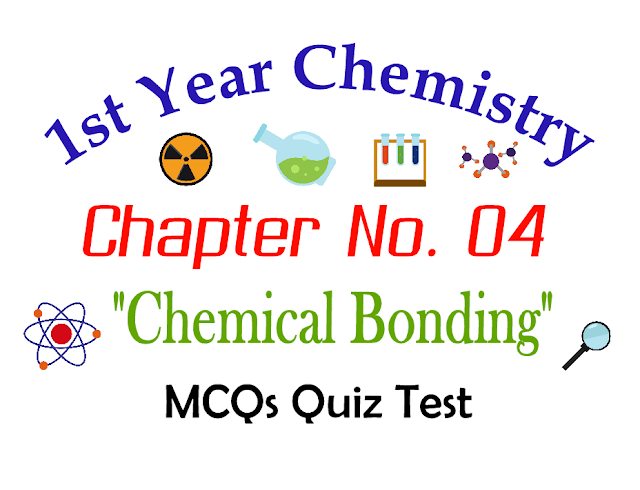# 1st Year Chemistry Chapter 04 Chemical Bonding MCQs Quiz Test

If you are looking MCQs for 1st year Chapter Four "Chemical Bonding" MCQs Question Answers with solutions you are here on right website. On this Page you will learn Chapter Four objective type solved Questions with solutions. All of these chapter wise first Year Chemistry question answers are helpful for upcoming exams and tests preparations. These MCQs are also helpful for entry tests.First Year Chemistry Chemical Bounding MCQs Quiz Test

## You will learn in this Chapter:

• Chemical Bond
• Atomic Sizes
• Ionization Energy, Electron Affinity and Electronegativity
• Lewis Concept
• Theories of Covalent Bond
• Atomic Orbit Hybridization
• Molecular Orbit Theory
• Bond Energy, Bond length and Dipole Moment

Q.1: Oxy-acids of halogens has the co-ordinate covalent bond between?
1. Hydrogen and oxygen
2. Hydrogen and hydrogen
3. Halogens and oxygen
4. Oxygen and oxygen
C
Q.2: Which of the following has a perfect triangular structure?
1. CO2
2. NO
3. PH3
4. SO3
D
Q.3: Limitation of valance bond theory (VBT) is that?
1. It doesn’t address the shapes of the molecules
2. It doesn’t involve the criteria of bond formation
3. It doesn’t involve the concept of valency
4. None of the above
A
Q.4: End to end overlapping of orbitals give rise to the formation of?
1. Sigma bond
2. Metallic bond
3. Pi bond
4. Coordinate covalent bond
A
Q.5: Side by side overlapping of orbitals gives rise to the formation of?
1. Sigma bond
2. Metallic bond
3. Pi bond
4. Ionic bond
C
Q.6: In sp3 hybridized orbital, the s character is?
1. 25%
2. 75%
3. 50%
4. 100%
A
Q.7: Dipole moment gives the information about?
1. % ionic character
2. The geometry of the molecules
3. Bond angles
4. All of the above
D
Q.8: Bonding affects properties like?
1. Solubility
2. Reaction kinetics
3. Melting, boiling points, and isomerism
4. All of these
D
Q.9: In sp2 hybridized orbital, the s character is-------.?
1. 11.11%
2. 33.33%
3. 22.22%
4. 44.44%
B
Q.10: An ionic compound A+B is most likely to form when?
1. The ionization energy of A is high and electron affinity of B is low
2. The ionization energy of A is low and electron affinity of B is high
3. Both the ionization energy and the electron affinity of A and B are high
4. Both the ionization energy and the electron affinity of A and B are low
B
Q.11: The bond order of nitrogen molecule and neon?
1. 2 and 1
2. 3 and 0
3. 0 and 3
4. 2 and 0
B
Q.12: Which of the following blocks will have comparatively higher electron affinity??
1. s block
2. f block
3. p block
4. d block
C
Q.13: Which one of the following has the maximum number of sp2 carbon atoms?
1. Benzene
2. Methane
3. CCl4
4. Ethene
A
Q.14: The process in which the orbitals of different energies and shape mix with each other to give equivalent hybrid orbitals is called?
1. Dissolution
2. Hybridization
3. Resonance
4. Ionization
B
Q.15: The structure of water is?
1. Trigonal
2. Angular
3. Linear
4. Tetragonal
B
Q.16: In a crystal, cations and anions are held together by?
1. Sharing of electrons
2. Nuclear forces
3. Electrostatic forces
4. Electrons
C
Q.17: Ionic compounds are obtained by the combination of groups?
1. 3 and 5
2. 1 and 7
3. 2 and 5
4. 4 and 8
B
Q.18: In a double bond connecting 2 atoms, there is a sharing of?
1. 1 electron
2. 2 electrons
3. 3 electrons
4. two-electron pairs
D
Q.19: F2 molecule is formed by the overlap of?
1. s-s orbital
2. s-p orbital
3. p-p head-on overlapping of orbitals
4. p-p parallel overlapping of orbitals
C
Q.20: Which of the following compounds has a non-directional bond??
1. BF3
2. KBr
3. Mg CO3
4. NF3
B
Q.21: The structure of CrO-24 is?
1. Tetrahedral
2. Octahedral
3. Cubic
4. Triclinic
A
Q.22: Number of electrons shared in SF6 molecule is-------.?
1. 4
2. 6
3. 8
4. 12
D
Q.23: The following molecules has a linear structure except??
1. CS2
2. SO2
3. CO2
4. BeCl2
B
Q.24: Which of the following species has lone pair of electrons on the central atom??
1. CH4
2. NH+4
3. PCl5
4. PCl3
D
Q.25: The shape of H3O+ is?
1. Tetrahedral
2. Angular
3. Pyramidal
4. Trigonal planar
C
Q.26: Which pair has trigonal planar geometry??
1. NH3, PH3
2. H2O, C2H2
3. BF3, AlH3
4. CO2, SO2
C
Q.27: Which of the following statements is not correct??
1. The double bond is stronger than the single bond
2. σ bond is stronger than π bond
3. The triple bond is shorter than a double bond
4. σ bond is weaker than π bond
D
Q.28: A molecule in which the distance between two carbon atom is largest is---------.?
1. Ethane
2. Ethene
3. Ethyne
4. Benzene
A
Q.29: All the metals shine when they are freshly cut. The reason is?
1. The conductivity of the metal is increased
2. The process of cutting gives energy to the metal atoms
3. The electron becomes less delocalized according to valance bond theory
4. The electrons are excited at higher energy levels and emit the photons when they fall back
D
Q.30: Total number of valance electrons of a nitrogen atom in an ammonium ion is?
1. 6
2. 10
3. 8
4. 16
C
Previous Post
Next Post